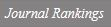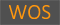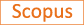Multiplicity of weak solutions to non-local elliptic equations involving the fractional p(x)-Laplacian

Title
Multiplicity of weak solutions to non-local elliptic equations involving the fractional p(x)-Laplacian
Authors
Lee, Jun IkKim, Jae-MyoungKim, Yun-HoLee, Jongrak
Ewha Authors
Issue Date
2020
Journal Title
JOURNAL OF MATHEMATICAL PHYSICS
ISSN
0022-24881089-7658Citation
JOURNAL OF MATHEMATICAL PHYSICS vol. 61, no. 1
Publisher
AMER INST PHYSICS
Indexed
SCI; SCIE; SCOPUSDocument Type
Article
Abstract
This paper is devoted to study the several existence results of a sequence of infinitely many solutions to the nonlocal elliptic problem involving the fractional p(x)-Laplacian without assuming the Ambrosetti and Rabinowitz type condition. The strategy of the proof for these results is to approach the problem variationally by using the fountain theorem and the dual fountain theorem. In addition, we prove that the sequence of weak solutions becomes bounded solutions. Published under license by AIP Publishing.
DOI
10.1063/1.5111786
Appears in Collections:
연구기관 > 수리과학연구소 > Journal papers
Files in This Item:
There are no files associated with this item.
Export
RIS (EndNote)
XLS (Excel)
XML